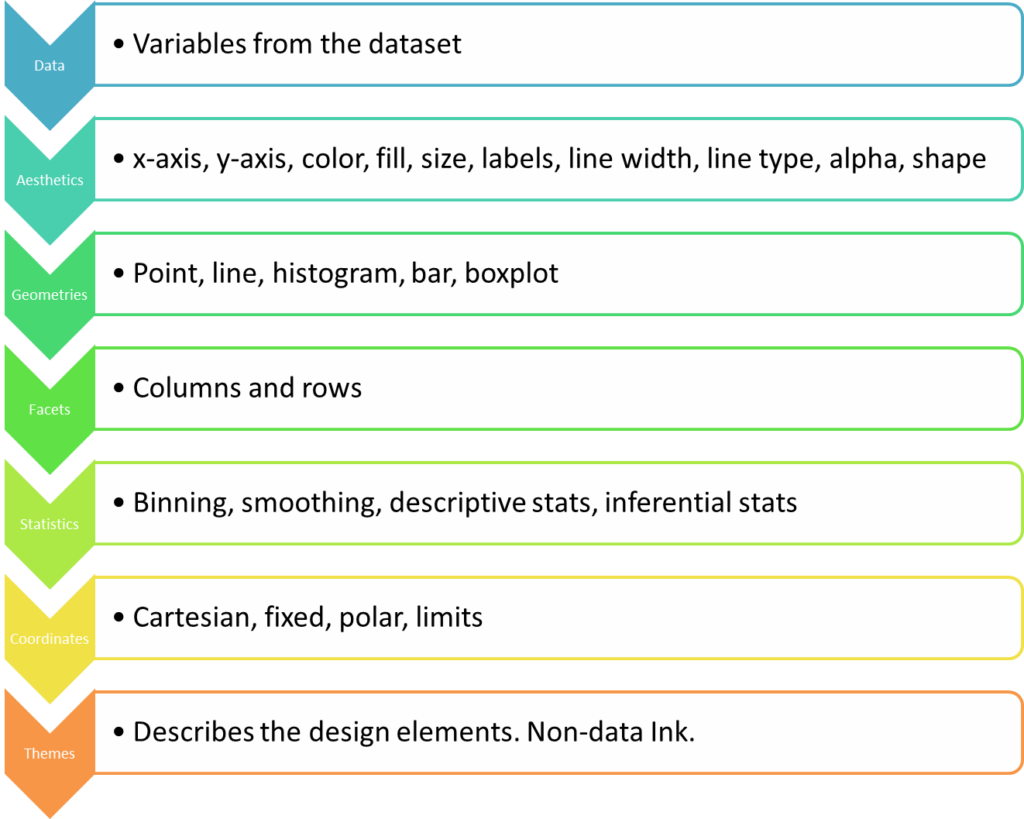# Grammar of Graphics in ggplot

We know that the grammar of a language is a set of rules that explain how words are used in a language to form meaningful sentences. Similarly, grammar of graphics defines rules for structuring mathematics and aesthetic elements into a meaningful graph, i.e., graphics are built based on an underlying grammar.

The grammar of graphics in ggplot is a plotting framework developed by Leland Wilkinson in his book 'Grammar of Graphics' published in 1999.

There are two important principles here:

1. Graphics are made of distinct layers of grammatical elements
2. Plots are built with appropriate aesthetic mappings to makes these plots meaningful

In brief, the grammar tells us that a statistical graphic is a mapping from data to aesthetic attributes (colour, shape, size) of geometric objects (points, lines, bars). The plot may also contain statistical transformations of the data and is drawn on a specific coordinate system

From ggplot2 book

### Components of Grammar of Graphics

There are 7 grammatical elements in ggplot2 with 3 being essential. The following table summarizes these elements:

ElementDescription
DataThis is the dataset being plotted containing the variables to be plotted on the graph.This is an essential element.
AestheticsAesthetics refers to the scales on which we map our data. For example, we will map one variable to the x-axis, and another to y-axis. We may use to differentiate different attributes by color. Some common aesthetics to consider are axis, shape, size and color.This is an essential element.
GeometriesGeom refers to the actual visual elements used for the data in the plot, such as points, lines, and bars.This is an essential element.
FacetsFaceting refers to splitting the data into multiple subsets and then displaying plots for the specific subsets in a panel. Such plots are also called small-multiple plots. The facet approach partitions a plot into a matrix of panels. Each panel shows a different subset of the data.This is an optional element.
StatisticsThis refers to representing statistical information about the data, such as mean and variance, to help in understanding the data.This is an optional element.
CoordinatesThis refers to the space on which the data is plotted (E.g., Cartesian coordinates). Most popular graphs such as line and bar charts are drawn using Cartesian coordinates.This is an optional element.
ThemesThemes are used to change the appearance of non-data elements. Themes enable you to design with a particular visual identity using fonts, colours and other design elements.This is an optional element.

It is useful to think of plots made up of layers. A layer can be thought of as consisting of the data, a mapping of data to aesthetics, and a geometry to visually display the data. Sometimes you can also use additional parameters to customize the display.

The grammar of graphics is implemented in R using the ggplot2 package. Essentially we develop plots by layering graphical elements on top of each other and use aesthetic mappings to refine our visualizations.

The following diagram provides an Ariel view of choices we will make while defining each layer in a plot. So, when we are making a plot with ggplot2, we are making choices from these items (Note: These are examples of choices. there are more choices that are not shown.)

In the next few lessons, we will learn about how we can create a variety of meaningful data visualizations using ggplot2 by implementing these 7 layers of grammar of graphics.